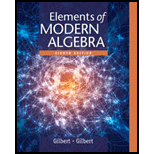# For the given f : Z → Z , decide whether f is onto and whether it is one-to-one. Prove that your decisions are correct. a. f ( x ) = { x 2 if x is even 0 if x is odd b. f ( x ) = { 0 if x is even 2x if x is odd c. f ( x ) = { 2 x + 1 if x is even x + 1 2 if x is odd d. f ( x ) = { x 2 if x is even x − 3 2 if x is odd e. f ( x ) = { 3 x if x is even 2 x if x is odd f. f ( x ) = { 2 x − 1 if x is even 2x if x is odd### Elements Of Modern Algebra

8th Edition
Gilbert + 2 others
Publisher: Cengage Learning,
ISBN: 9781285463230### Elements Of Modern Algebra

8th Edition
Gilbert + 2 others
Publisher: Cengage Learning,
ISBN: 9781285463230

#### Solutions

Chapter
Section
Chapter 1.2, Problem 11E
Textbook Problem

## Expert Solution

### Want to see the full answer?

Check out a sample textbook solution.See solution

### Want to see this answer and more?

Experts are waiting 24/7 to provide step-by-step solutions in as fast as 30 minutes!*

See Solution

*Response times vary by subject and question complexity. Median response time is 34 minutes and may be longer for new subjects.